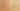AssignmentNotExpression

Misconception:

An assignment, like a = b, is not an expression but a statement. It thus cannot be used in a context where an expression is expected. E.g., it is incorrect to write min(a = 1, b = 2) or if (a = true) {...} or a = b = c.Incorrect

An assignment a=b is not an expression

Correct

An assignment a=b is an expression and thus produces a value

CorrectionHere is what's right.

In Java, an assignment is an expression. Thus, it can appear as a subexpression in a larger expression.

Common Uses of Assignments as Subexpressions

One use case is to assign a value to multiple variables in a single statement:

int x;
int y;
x = y = 1;
x = (y = 1); // same

The corresponding expression tree looks as follows. The left-hand side of each assignment operator provides the variable into which to store the value provided by the right-hand side.

Another use case is when reading lines from a file. Here the BufferedReader.readLine() method returns a String with the read line, or null if the end of the stream is reached. The while-loop condition is an expression that tests for this null, but that also has the side effect of assigning the read line (or null) to a variable to be used in the loop.

String line;
while ( (line = br.readLine()) != null) {
// handle line
}

OriginWhere could this misconception come from?

Students may have prior knowledge in a language where assignments indeed are statements.

ValueHow can you build on this misconception?

In some languages an assignment is a statement but not an expression. Thus, a student who holds this Java misconception may think not unlike the designer of such languages.

For example, Python uses = for assignment statements. In version 3.8 it introduced the assignment operator (:=) to also support assignment expressions.

x = 1                           # Assignment statement

while chunk := file.read(1024): # Assignment expression
...

Java